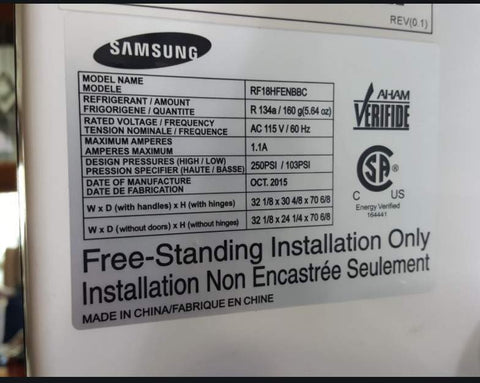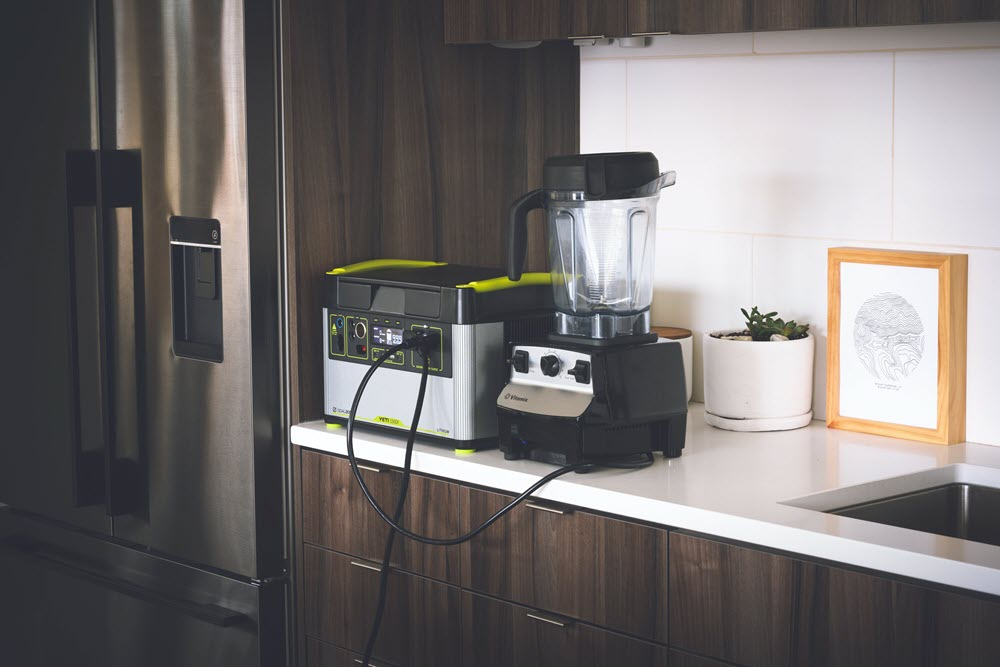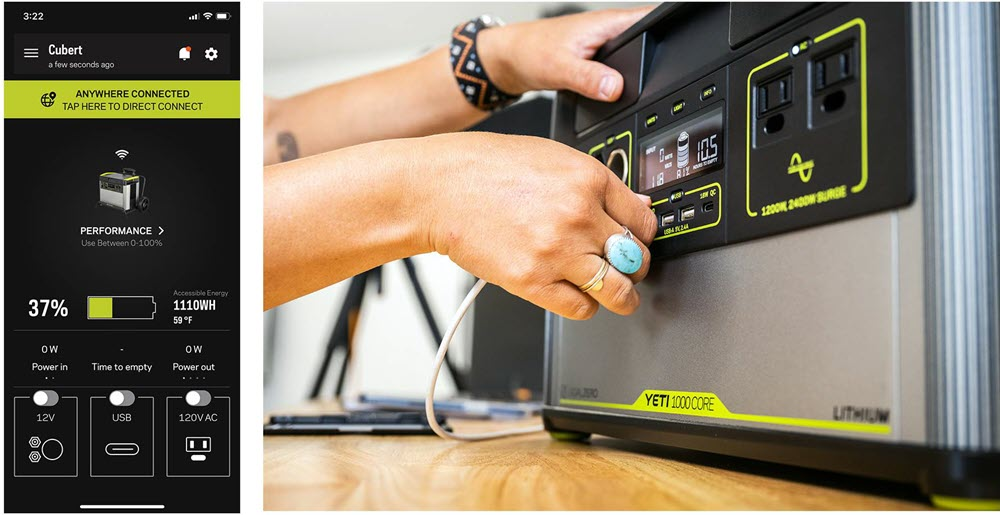# BACK TO BASICS | WHAT IS A WATT HOUR?

As you scroll through our product pages, you may notice that we use Watt Hours to explain the capacity, or amount of charge, stored in our batteries. Watts and Watt Hours may be familiar terms, but an unfamiliar concept. While they are related, they are not the same – and we’re here to help clear up the mystery. Let’s start with the basics!

## WHAT IS A WATT?To better understand Watt Hours, we must first explain a Watt. A Watt is a unit of measurement for power. Power is the rate at which energy flows. If you think of Watts as a measurement of flow, it’s easy to determine if a device will need a big flow or small flow to work. The higher the wattage requirements, the bigger the flow needed in order for the device to function.

Let’s say rolling blackouts are common in your area during the summer and you need to determine how much power is required to keep your fridge running. Ever notice the sticker on the inside wall of your fridge with the model information? That same sticker will often show your fridge’s power draw, as will the user manual. If you can only find the voltage and amperage, a quick calculation will tell you how many watts your fridge needs.

### HOW ARE WATTS CALCULATED?

Once you find the number of volts and amps, it’s easy to determine how many Watts your fridge uses. Watts are calculated using the following equation:

Volts x Amps = Watts

Let’s say you’ve figured out that your fridge requires 15 Volts and 3.5 Amps, resulting in a maximum power draw of 52.5 Watts.

15 Volts x 3.5 Amps = 52.5 Watts

Conversely, you can manipulate this formula based on the information you can find. If, for example, Amps are not listed, you can divide the wattage by Volts. In the US, all regular outlets are 120 Volts, so you can use the equation:

Watts / Volts = Amps

A quick Internet search can also give you an estimate of the average voltage of most devices, so don’t worry if your product does not have Amps or Volts listed.

Note: Because varying factors – like ambient home temperature, fridge efficiency, how long and often the fridge is opened – can all cause fluctuations, we use Energy Star’s Standard Test Conditions (STC), to help generalize our products’ runtimes.

#### WHAT IS A WATT HOUR?

Watt Hours are a unit of measurement for energy. Energy is the total sum of power over a period of time – in other words, a way of measuring capacity. Remember: capacity refers to the amount of charge stored in a battery. In this case, we use the time period of 1 hour. So, what’s the difference between Watts and Watt Hours? Watts measure the rate of power at a moment in time, and Watt Hours measure amount of power for a specific period of time.

##### HOW ARE WATT HOURS CALCULATED?

When finding the capacity of a battery, the Watt Hours are calculated using the equation:

Nominal Voltage x Amp Hours = Watt Hours

The Yeti 1500X’s battery has a nominal voltage of 10.8 Volts, and if we multiply that by its 140.4 Amp Hours, we arrive at 1516 Watt Hours.

10.8 Volts x 140.4 Amp Hours = 1516 Watt Hours

Note: Nominal Voltage is the “average” Voltage in the operating range of a battery (e.g., a standard lead acid battery has a nominal Voltage of 12 Volts).

###### SO HOW LONG CAN YOU POWER YOUR FRIDGE?1 Watt Hour is equal to 1 Watt of average power flow over the course of an hour. 1 Watt over 5 hours would be 5 Watt Hours of energy. So, your 50 Watt fridge powered by a 1500 Watt Hour battery (like the Yeti 1500X) would last, on paper, 30 hours. It’s important to note this is before things like line loss and power conversion inefficiencies take place.

1500 Watt Hours / 50 Watt Fridge = 30 Hours

While we’re using a 50 Watt fridge as a number to make our math simple, the reality is all fridges are different in their power draw. So, what does this mean when using the Yeti 1500X in a real-life application? If we take into consideration the factors that affect runtimes, it is safe to assume that in an emergency, the Yeti 1500X will keep your food cold for around 22 hours.Similar to your car’s “trip” counter, our Yeti portable power stations have a home display equipped with a resettable Watt Hour tracker that counts battery output. Tracking energy usage is also simple with our app that reflects every Watt Hour flowing in and out of your Yeti in easily digestible graphs. Both the power stations and app also allow you to track how many hours you have left in a charge, as well as how many hours until your unit is fully charged.

Watt Hours provide us with a metric by which we can both communicate battery capacity, as well as compare different battery sizes. Once you have a basic understanding of Watt Hours, you can better gauge how many hours one of our GZ products will keep your devices charged.